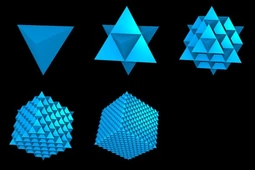64 tetrahedron grid(1+2+8 Metatron's Cube/7 tetractys/fruit of life/tetragrammaton/tetractys/star tetrahedron/tree of life)

The Flower of Life and the 64 Tetrahedron grid: the mother and father of the geometry of the fabric spacetime

64 tetrahedrons: the fundamental seed geometry of the fabric of the vacuum of spacetime itself

## 64 tetrahedron grid

I Ching is one of the world’s oldest texts, dated to 1000 years BC, and it is said to have origins even further back in time. The text describes the laws of nature through the union of polarities and its diagram consists of 64 hexagrams representing various points of balance between Yin and Yang – negative and positive energy. When we put all opposing polarized hexagrams together they form the lines of a 64 and a 512-tetrahedral matrix, which is the necessary geometry to describe the perfect balance and fractal recursion of space-time. The fact that the diagram depicts two iterations of the fractal – 64 and 512 tetrahedrons – is no coincidence. As we have seen in the Fractal-Holographic Universe it is precisely this difference of vector density in the vacuum geometry which is the source of the universal torque, centrifugal forces and thus visible manifestation. The density of vectors required to form symmetrical stability of the infinite vacuum energy steadily increases towards higher density as we move into deeper resolutions of the fractal. This creates relative gradients of energy density, from infinitely dense to infinitely un-dense, and it is precicely these differences that drives space-time torque at all levels, from atom to universe, in the same way that small differences in pressure and temperature in the weather systems on earth drives enormous hurricanes.The hexgrams of the I Ching depicts a three-dimensional fractal tetraedral array with two recursive iterations from 64 to 512 tetrahedron-tetrahedron. This is nature’s geometry. The difference in vector density is the source of rotation.

I this manner a continuously imploding fractalized space-time creates universal torque and organizes matter/energy in harmonically embedded rotating tori; galaxies with billions of stars, stars with dozens of planets, planets with rotating hemispheres etc. The systems  appear to us as atoms, bio-entities, stellar systems and vast galaxies but they are merely aspects of the same cosmic vacuum singularity albeit in differentiated and localized form.Galaxy’s rotation is driven by a central singularity – the torque applied by the spacetime increasing density towards the infinitesimal.

## flower of life

if you put a sphere around each tetrahedron of the 64 tetrahedron grid you get the 3d flower of life

## Metatron's cube

the 64 tetrahedron grid is Metatron's cube

### fruit of life

the fruit of life encodes Metatron's Cube which encodes the 64 tetrahedron grid

### fruit of life superstring theory

the fruit of life encodes 4 equilateral triangle's which are 3,6,9(energy) and encode all frequencies which are dimensions and the frequencies are multidimensional polygons

if you add up all these frequencies you get -1/12the fruit of life encodes 4 equilateral triangle's which are 3,6,9(energy) and encode all frequencies which are dimensions and the frequencies are multidimensional polygons if you add up all these frequencies you get -1/12

## Quantum energy structure

The structure of the Xen energy that makes up existence is called a Morphogenetic Field and its shape is the 64 tetrahedron grid

## Sri Yantra

64 tetrahedron grid=Sri Yantra

## tree of life and 64 tetrahedron grid

the 64 tetrahedron grid is made out of 8 tree of life's

### tree of life and star tetrahedron

the 64 tetrahedron grid is made out of 8 star tetrahedron's meaning that the tree of life is the star tetrahedron

## infinite tetrahedron grid

the infinite tetrahedron grid is made out of infinite 64 tetrahedron grid's

### infinite fractal fruit of life

the infinite fractal fruit of life encodes the infinite tetrahedron grid

## E8 lie group and 64 tetrahedron grid

(64 tetrahedron grid)=(E8 lie group)

The E8 Lie group has applications in theoretical physics and especially in string theory and supergravity. E8×E8 is the gauge group of one of the two types of heterotic string and is one of two anomaly-free gauge groups that can be coupled to the N = 1 supergravity in ten dimensions. E8 is the U-duality group of supergravity on an eight-torus (in its split form).

One way to incorporate the standard model of particle physics into heterotic string theory is the symmetry breaking of E8 to its maximal subalgebra SU(3)×E6.

In 1982, Michael Freedman used the E8 lattice to construct an example of a topological 4-manifold, the E8 manifold, which has no smooth structure.

Antony Garrett Lisi's incomplete "An Exceptionally Simple Theory of Everything" attempts to describe all known fundamental interactions in physics as part of the E8 Lie algebra

## 444,84,7 tetractys and the 64 tetrahedron grid

• There are 84(7 tetrahedral tetractys, there are 84 yods in the cosmic tetractys which corresponds to the cosmic tree of life) vesica piscies in the 3D flower of life that encodes the 64 tetrahedron grid.
• 7 tetrahedral tetractys(3D 7 tetractys(1+2+3+4+5+6+7=28))=84=44:4(8:4)=444=64(64 tetrahedron grid)